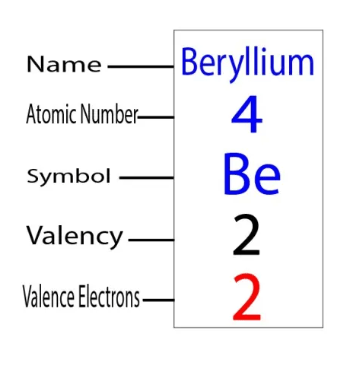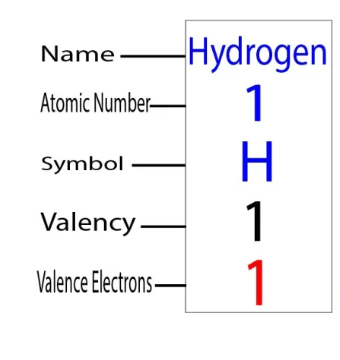Valence electrons
How many valence electrons does Boron have?
01.5k.
Boron (B) is fifth element on the periodic table. The symbol for Boron is “B”. Boron is involved in the formation bonds via valence electrons.Valence electrons
How many valence electrons does Silicon have?
01.5k.
Silicon is the 14th Element of the periodic table. Silicon is a semiconductor material. Its symbol is “Si”. Silicon is involved in the formationValence electrons
How many valence electrons does Beryllium have?
01.3k.
The symbol for Beryllium, the fourth element on the periodic table, is “Be”. Beryllium is involved in the formation bonds via valence electrons.Valence electrons
How many valence electrons does Scandium have?
11.3k.
Scandium is the 21st element on the periodic table. Scandium is the element in group 3 and its symbol, ‘Sc’. Scandium is an element of a sideValence electrons
How many valence electrons does Lithium have?
01.3k.
Lithium (Li) is third in the periodic table. It is also the 2nd element of group-1. The standard atomic weight of lithium is 6.938. Its symbol is “Valence electrons
How many valence electrons does Helium have?
01.1k.
Helium is the second element on the periodic table, and the first element of Group-18. Helium is represented by the symbol “He”Valence electrons
How many valence electrons does Hydrogen have?
0996
Only one electron is needed for a hydrogen atom. Hydrogen is represented by the symbol “H”. Hydrogen is the most abundant chemical element